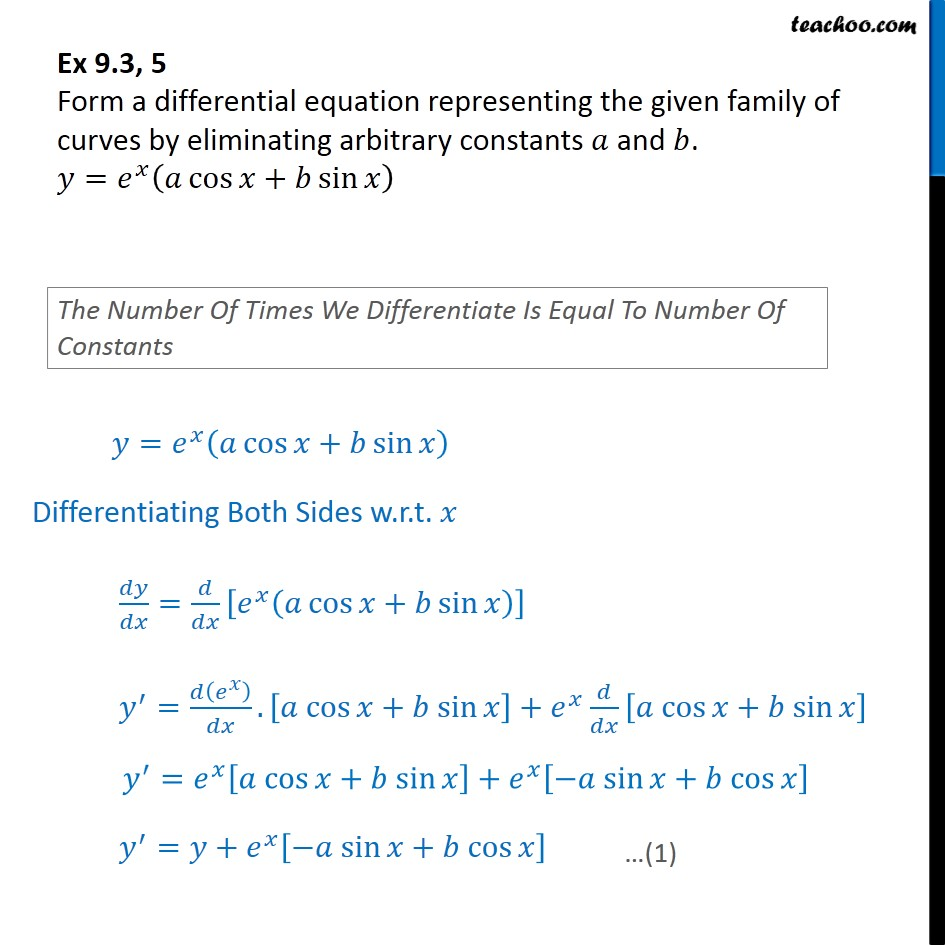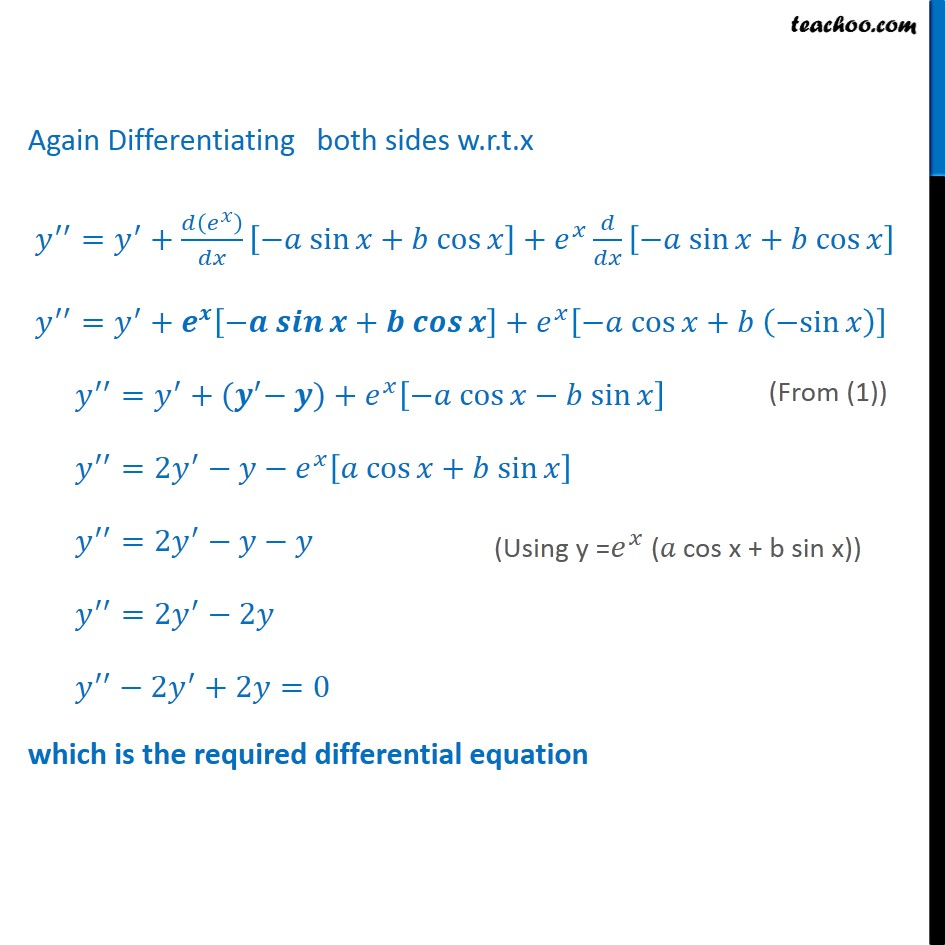1. Chapter 9 Class 12 Differential Equations
2. Serial order wise
3. Ex 9.3

Transcript

Ex 9.3, 5 Form a differential equation representing the given family of curves by eliminating arbitrary constants 𝑎 and 𝑏. 𝑦=𝑒^𝑥 (𝑎 cos⁡〖𝑥+𝑏 sin⁡𝑥 〗 ) Again Differentiating both sides w.r.t.x 𝑦^′′=𝑦^′+𝑑(𝑒^𝑥 )/𝑑𝑥 [−𝑎 sin⁡𝑥+𝑏 cos⁡𝑥]+𝑒^𝑥 𝑑/𝑑𝑥 [−𝑎 sin⁡𝑥+𝑏 cos⁡𝑥] 𝑦^′′=𝑦^′+𝒆^𝒙 [−𝒂 𝒔𝒊𝒏⁡𝒙+𝒃 𝒄𝒐𝒔⁡𝒙]+𝑒^𝑥 [−𝑎 cos⁡𝑥+𝑏 (−sin⁡𝑥)] 𝑦^′′=𝑦^′+〖(𝒚〗^′− 𝒚)+𝑒^𝑥 [−𝑎 cos⁡𝑥−𝑏 sin⁡𝑥] 𝑦^′′=2𝑦^′−𝑦−𝑒^𝑥 [𝑎 cos⁡𝑥+𝑏 sin⁡𝑥] 𝑦^′′=2𝑦^′−𝑦−𝑦 𝑦^′′=2𝑦^′−2𝑦 𝑦^′′−2𝑦^′+2𝑦=0 which is the required differential equation

Ex 9.3